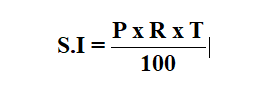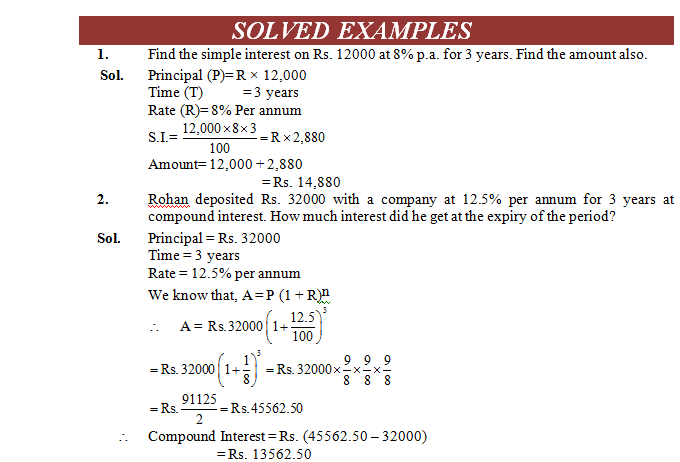# Simple Interest

## Important Terms

The money borrowed or lent out for a certain period of time is called the principal or sum.

Interest : The additional money paid by the borrower in lieu of the money used by him is called interest. Amount :The total money paid back to the lender is called amount.

Amount = Principal + Interest

Rate :Interest on Rs. 100 for 1 year is called rate per cent per annum (abbreviated as rate % p.a.) Thus, if rate = 9% per annum, then it means that the interest on Rs. 100 for 1 year is Rs. 9.

Simple Interest :If interest is reckoned uniformly on the original principal throughout the loan period then the interest is called simple interest, abbreviated as S.I.

### Formula :

Let Principal = Rs. P, Rate = R% per annum and Time = T years.

Then, we have